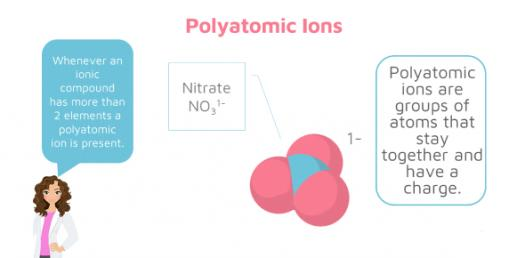# What Do You Know About Polyatomic Ions? Trivia Quiz

11 Questions | Total Attempts: 15SettingsWhat do you know about polyatomic ions? Whenever an ionic compound has more than two elements, then a polyatomic ion is present. According to our classes, we got to understand that a polyatomic ion is made up of more than one atom. Get to review all you know about these ions by taking this trivia quiz. All the best!

Related Topics
• 1.
What is H30+?
• A.

Hydronium ion

• B.

Phosphate ion

• C.

Hydrogen ion

• D.

Hydroxide ion

• 2.
What is the formula for an Acetate ion?
• 3.
CO32-
• A.

Hydroxide ion

• B.

Bicarbonate ion

• C.

Carbonate ion

• D.

Cation

• 4.
Phosphate ion formula.
• A.

OH-

• B.

PO43-

• C.

NH4+

• D.

Anion

• 5.
HCO3- is the formula for what polyatomic ion?
• 6.
What is the formula for a Hypochloride ion?
• 7.
NH4+
• A.

Ammonium ion

• B.

Phosphate ion

• C.

Hypochloride ion

• D.

Acetate ion

• 8.
What is the formula for a bicarbonate ion
• A.

H3O+

• B.

NH4+

• C.

HCO3-

• D.

PO43-

• 9.
What is the formula for a Hydroxide ion?
• 10.
OCl-
• A.

Phosphate ion

• B.

Hypochloride ion

• C.

Chlorine

• D.

Hydroxide